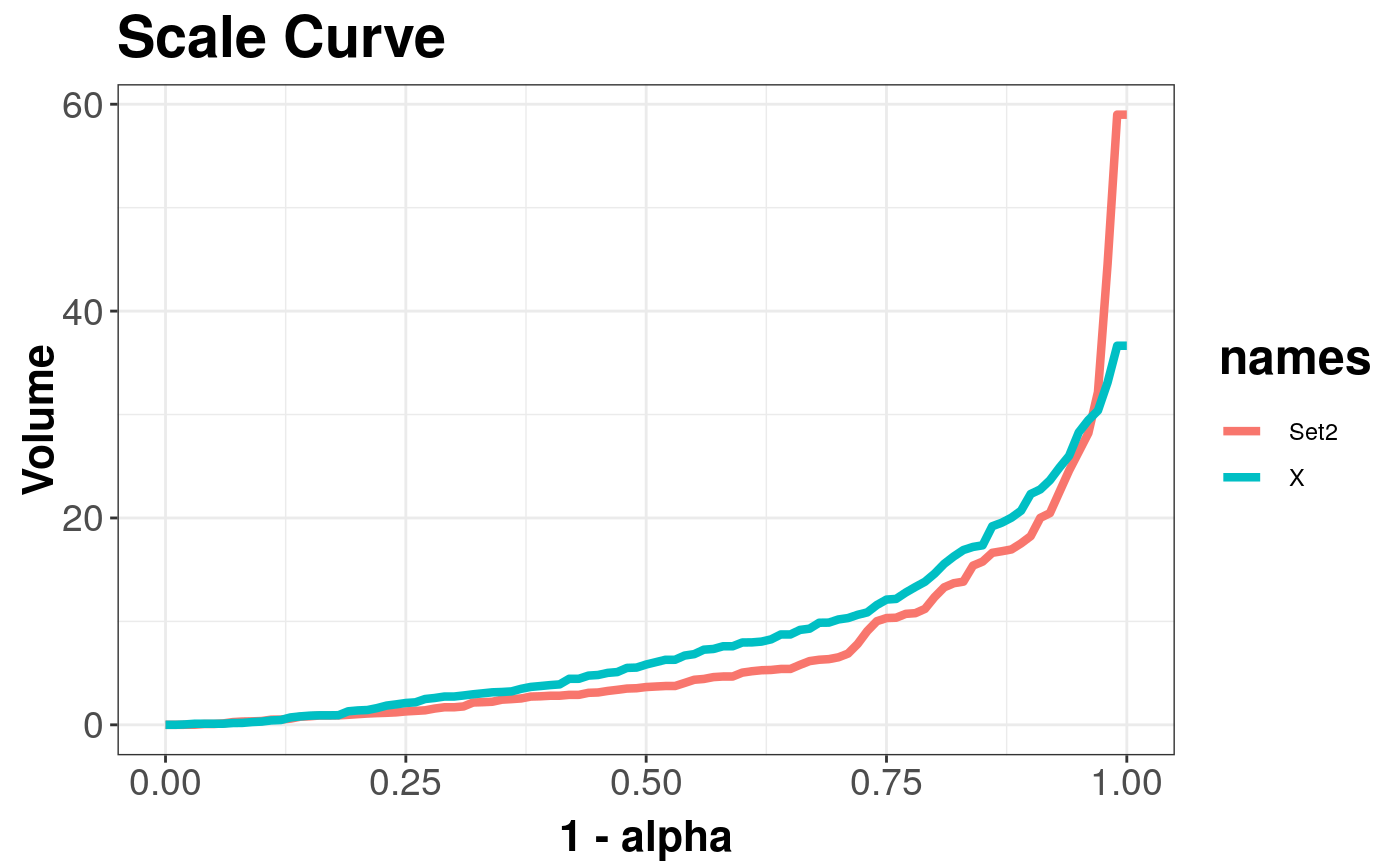ScaleCurve is a class that stores results of scaleCurve function.

## Details

ScaleCurve intherits behviour from numeric vector, so raw values of ScaleCurve can be accessed via as.numeric(...).

The mechanism of creating plots with multiple curves is shown in DepthCurve-class (same mechanism is applied for AsymmetryCurve).

## Examples

library(mvtnorm)
x <- mvrnorm(n = 100, mu = c(0, 0), Sigma = 2 * diag(2))
y <- rmvt(n = 100, sigma = diag(2), df = 4)
s1 <- scaleCurve(x, depth_params = list(method = "Projection"))
s2 <- scaleCurve(y, depth_params = list(method = "Projection"), name = "Set2")

sc_list <- combineDepthCurves(s1, s2) # Add one curve to another

plot(sc_list) # Draw plot with two curvesz <- mvrnorm(n = 100, mu = c(0, 0), Sigma = 1 * diag(2))
s3 <- scaleCurve(z, depth_params = list(method = "Projection"))
plot(combineDepthCurves(sc_list, s3)) # Add third curve and draw a plot#> Warning: Names in DepthCurveList are not unique!